# Robust statistics

Jump to: navigation, search

The branch of mathematical statistics concerned with the construction and investigation of statistical procedures (such as parameter estimators and tests) that still behave well when the usual assumptions are not satisfied. For instance, the observations may not follow the normal (Gaussian) distribution exactly, because in practice the data often contain outliers (aberrant values) which are caused by transcription errors, misplaced decimal points, exceptional phenomena, etc.

It has been known for many years that the classical procedures (such as the sample average) loose their optimality, and can in fact give arbitrarily bad results, when even a small percentage of the data are outlying. In such situations applied statisticians have turned to alternative methods (e.g. the sample median) which they knew to be less affected by possible outlying values. However, the intuitive notion of robustness was not formalized until the 1960's. The theoretical foundations of robust statistics have been developed in the three stages described below.

The first mathematical approach is due to P.J. Huber [a1], who found the solution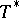to a minimax variational problem: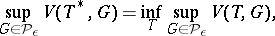where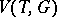denotes the asymptotic variance of an estimatorat a distribution. The set(for some) consists of all mixture distributions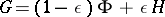, whereis the standard normal distribution andis arbitrary (e.g., the Cauchy distribution). In the framework of a univariate location parameter, the solutionhas come to be known as the Huber estimator. For a survey of this work see [a2].

The second approach was based on the influence function [a3]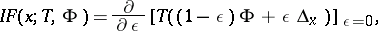where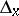is the probability distribution which puts all its mass in the point. The influence function describes the effect of a single outlier aton (the asymptotic version of) the estimator. In this approach one obtains optimally robust estimators and tests by maximizing the asymptotic efficiency subject to an upper bound on the supremum norm of the influence function. Unlike the minimax variance approach, it can also be applied to multivariate problems. The main results of this methodology are found in [a4].

The third approach aims for a high breakdown point. The breakdown point of an estimator is the largest fraction of arbitrary outliers it can tolerate without becoming unbounded. This goes beyond the second approach, where only the effect of a single outlier was considered. In linear regression analysis, the first equivariant high-breakdown estimator was the least median of squares [a5], defined by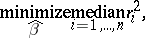where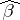is the vector of regression coefficients and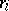is the residual of the-th observation. High-breakdown estimators also exist for other multivariate situations. A survey of these methods is given in [a6], which also describes applications.

At present (1990's), research is focussed on the construction of estimators that have a high breakdown point as well as bounded influence function and good asymptotic efficiency. The more recently developed estimators make increasing use of computing resources.